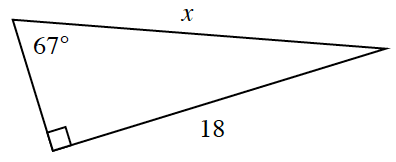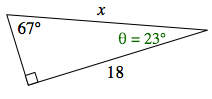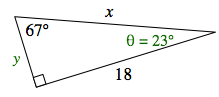### Home > CCG > Chapter 5 > Lesson 5.1.4 > Problem5-46

5-46.Estelle is trying to find $x$ in the triangle at right. She lost her scientific calculator, but luckily her teacher told her that $\sin23^\circ\approx0.391$, $\cos23^\circ\approx0.921$, and $\tan23^\circ\approx0.424$.

1. Write an equation that Estelle could use to solve for $x$.

Use the Triangle Angle Sum Theorem to calculate the missing angle, this means all the angles in a triangle total $180^\circ$Label added, third angle, theta = 23 degrees.

$67^\circ + 90^\circ + θ = 180^\circ$
$-157-157$
$θ = 23^\circ$

Place a "$y$" on the missing side. Write the trigonometric ratios for the angle, reference the Math Note box in Lesson 5.1.2 for extra help. Label added, short side is y.

$\sin23^\circ=\frac{y}{x}\ \ \ \ \cos23^\circ=\frac{18}{x}\ \ \ \tan23^\circ=\frac{y}{18}$Which equation can be used to solve for $x$?

${\cos 23^\circ=\frac{18}{x}}$

2. Without a calculator, how could Estelle find $\sin67^\circ$? Explain.

$\text{sin}(67^\circ)=\frac{\text{opposite}}{\text{hypotenuse}}=\frac{18}{x}$

$\text{sin}(67^\circ)=\frac{18}{x}=\text{cos}(23^\circ)$

$\cos23^\circ\approx0.921$

$\sin67^\circ=\cos23^\circ\approx0.921$